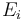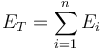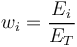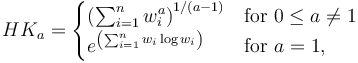# Hannah Kay Index

## Definition

For the purpose of measuring concentration (e.g., name, sector or geographic concentration), the Hannah-Kay Index is defined as the sum product of relative portfolio shares of the exposures raised to a desired exponent (power).

## Details

More precisely, if we have n exposures$E_i$ summing up to a total exposure of$E_T = \sum^{n}_{i=1} E_{i}$

where each exposure fraction is defined  as$w_{i} = \frac{E_i}{E_T}$

Then the Hannah-Kay index is defined as$HK_{a}= \begin{cases} \left(\sum_{i=1}^{n} w_{i}^{a}\right)^{1/(a-1)} & \mbox{for}\ 0 \leq a \neq 1 \\ e^{\left(\sum_{i=1}^{n} w_i \log w_{i}\right)} & \mbox{for}\ a=1, \end{cases}$

None

None

None

## Implementations

Open Source implementations of the Hannah-Kay index are available in